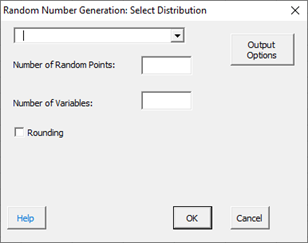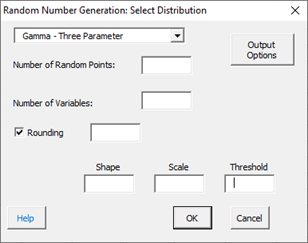# Random Number Generator HelpSPC for Excel generates random numbers for the following distributions:

• Exponential
• Exponential – Two Parameter
• Gamma
• Gamma – Three Parameter
• Largest Extreme Value
• Logistic
• LogLogistic
• LogLogistic – Three Parameter
• LogNormal
• LogNormal – Three Parameter
• Normal
• Smallest Extreme Value
• Weibull
• Weibull – Three Parameter

1. To access the random number generator, select  “Sample Tests” from the “Statistical Tools” panel in the SPC for Excel ribbon.

2. Select “Random Number Generator” and the input form below is shown.3. Select the distribution you want to use to generator random numbers (Gamma – Three Parameter in the example).4. Enter the number of random points you want generated.

5. Enter the number of variables you want random points generated for.

5. Enter the parameters for the distribution you selected.  The distributions are defined by one or more of four parameters:

• Location
• Shape
• Scale
• Threshold

6. Select OK and the random numbers are generated on a new worksheet.

7. You can change where the random numbers are generated by selecting the “Output Options” button. This will display the form below.You can select a cell on the current worksheet or keep the default option of a new worksheet.